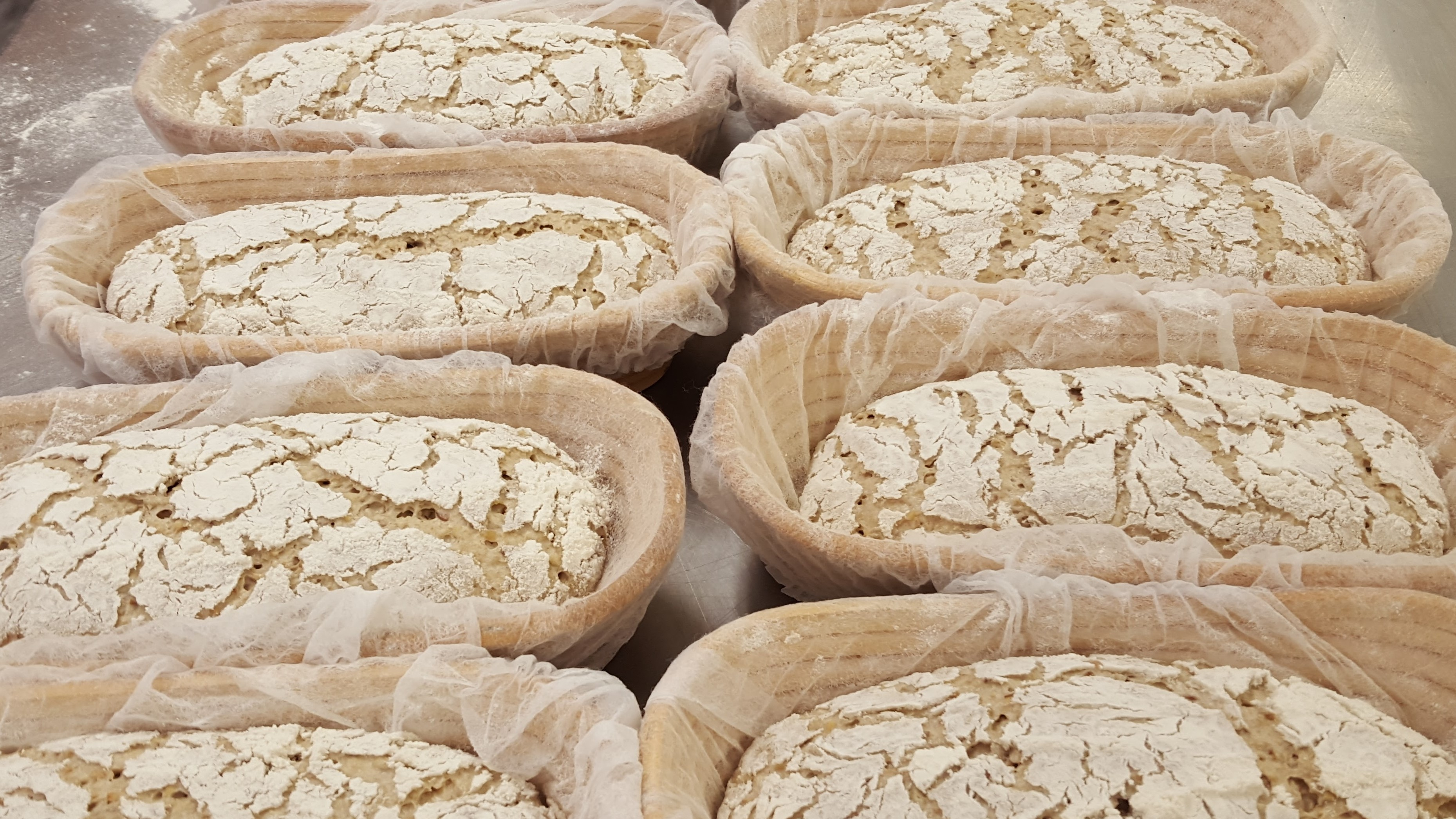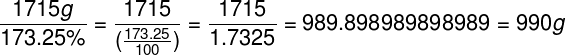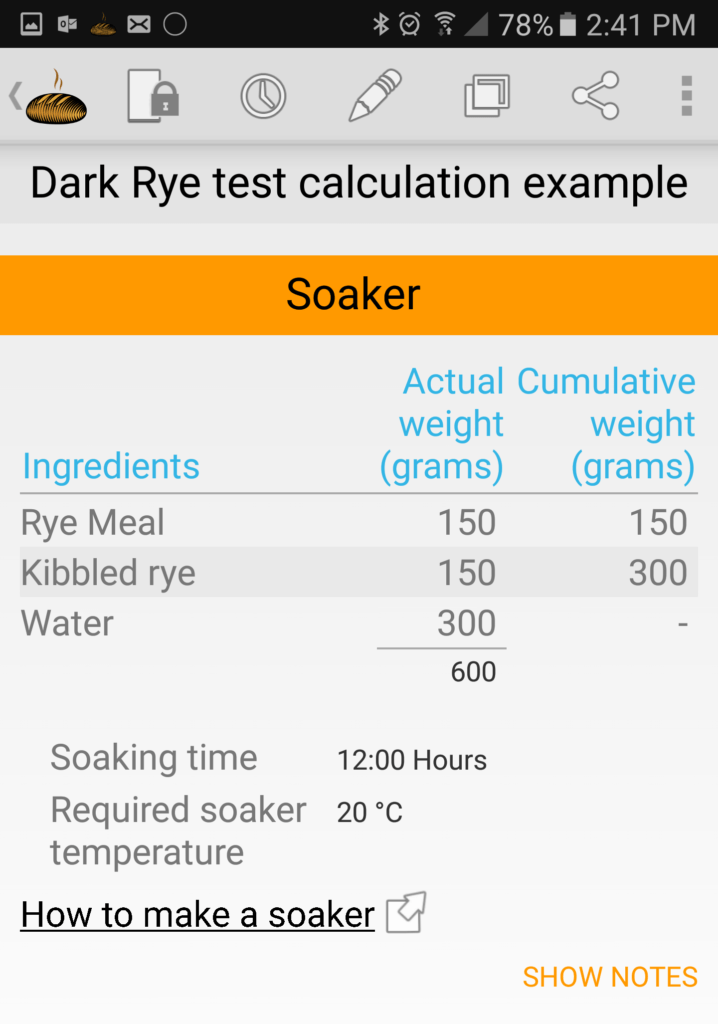Posted by on Jun 23, 2016 in Baking Tips, slider | 0 comments# The Bakers Percentage

Making bread isn’t the same as cooking, bread making is a straight forward process but it involves a combination of biochemical and physical processes by living organisms. This means it’s important to have a balanced formula as microorganisms are very sensitive to changes to their environment – in this case – bread dough. It also means ingredients at the right weights. I use the word “formula” for good reason. Most uninitiated in bread baking believe that the word ‘formula’ and ‘recipe’ are really the same. This is not the case in bread baking. There’s a subtle but important difference.

A recipe is a list of ingredients with weights or volumes (depending on how it’s written). It can also include methods and other instructions. The upshot is that it’s meant to be followed as is.

A formula however, is also a list of ingredients often with method included, but rather than just weights or volumes the ingredients are expressed as percentages (%).

Here’s an example:

Notice that the flour is considered 100% and all the other ingredients are a percentage of that 100. Another way of seeing it is that the flour is the ‘whole number’ and the other ingredients are the ‘decimals’ or ‘fractions’ of the whole. If you’re not familiar with this reasoning it’ll appear counter-intuitive to designate flour as 100%, since 100% means, the whole of the dough, not just one part of it. Yes, but that’s why it’s called the “bakers percentage”. Using the percentage the way bakers do enables us to use it to calculate yields with ease. It also allows bakers to immediately see the relationship each ingredient has to the flour and to each other. This is no trivial thing for bakers!

Lets go further:

• Lets say we want to make 7 baguettes/units that each weigh 245g

We will need to know how much dough we will require in total to make those units.

• 7   x   245g  = 1715g  (Required dough)

Next we will determine the flour required to make that specific amount of dough. This is where the bakers percentage is so useful.

If we take the example above as the formula for the 7 baguettes we calculate it this way…

• required dough ÷ total percentage = weight of flour
• 1715g ÷ 173.25%* = 990g (required flour)

*See total percentage in Fig. 1 above• Total required dough – divided by the total percentage – in this case 173.25% this gives us the flour weight of 990g.

NOTE: In any real life situation we can’t do anything perfectly and that includes weighing ingredients and dividing dough. So we round up the flour to account for variations and process losses. In this case we will round the flour weight to 1kg or 1000g. See the table below.

Note in the example above that we now have enough to make the required dough for our 7 baguettes. The beauty is that the ratios of the ingredients are the same no matter how much dough is required, either less or more.

Using this same method we can impose extra stages of dough prep. For example we can impose a sourdough or a yeasted preferment, or a soaker also within the same formula and still preserve the formula balance. See the table below.

In order to understand this formula we start by focusing on the Base%. This is where we start to understand the ratios, which are really the heart of the formula. Then we look at the imposed formula – SOURDOUGH  – within the base formula. It should be clear that everything that is in the dough, no matter how many sub-formulas we impose on the base formula, must come from the base formula.

In this example we have used 10kg – 10.000 – 100000g – flour weight so the percentages and weights are easily identifiable by the uninitiated.

In detail: the base weight is simple enough to understand and see. The sourdough formula is also simple enough but the ingredient weights are determined by the flour weight in the sourdough. The flour weight in the sourdough is dictated by the percentage of flour to be acidified (FTA). In this case it happens to be 30%. Hence 30% of the total flour is set aside 12 hours earlier to be fermented by the starter which infects the batch with the desirable microorganisms.

Therefore:

• total flour                   = 10.000
• FTA 30%                    =   3.000 (total flour to be acidified) In this sourdough the flour is divided into two types – Bakers flour and stoneground flour.
• bakers flour 80%               = 2.400
• stoneground flour 20%    = 0.600
• total FTA                             = 3.000

Added to this flour is water and starter.

• water 55% of 3.000         = 1.650
• starter 20% of 3.000       = 0.600

Total sourdough is the addition of all the ingredients excepting the starter which is removed from the sourdough at the point of maturity.

Note: at removal the starter has been cycled ready to infect another batch for further bread to be made. It will now be stored in the fridge until required.

To continue with this example the “actual” column shows the weights that assemble the final dough when the sourdough is added and then the balance of the final bread dough ingredients.

Note that the Bakers flour, stoneground, and water have been reduced in the “Actual” column when compared to the base weight column. The reason for this is that the ingredients from the base weight “borrowed” earlier to make the sourdough have come back to the final dough. Hence, those ingredients need to be reduced to maintain the correct amount in the final dough. Notice the total weight in both the base weight column and actual column are identical – 10.740kg. This indicates that the formula is balanced even though a sourdough sub-formula has been imposed.

Using the same principle any number of “imposed” stages/formulas from within the base weight can be made to effect any idiosyncratic result the Baker may require.

So to illustrate this, see the formula below, it not only incorporates a sourdough but also a soaker.

A soaker is a portion of the bread dough ingredients allowed to soak overnight to effect hydration and enzyme activity to produce maltose sugars as a result of diastatic activity .

So how does this tie in with Bread Boss? Bread Boss uses the same principles that I’ve shown here in our paper calculations. The differences are that much of the background figures are not shown because Bread Boss does the calculations for you. This is a significantly easier approach as it behaves much the same as a calculator with baking “rules” if you like. Of course Bread Boss also has the flexibility to allow you to override those rules but it will signal a warning in order to make the user aware.

So now lets see how Bread Boss displays the same formulas and the differences that may be apparent as an exercise in becoming familiar with the process.

Dark Rye showing the sourdough, soaker and final dough.I hope this helps explain some of how bakers use percentages to ensure their formulas are balanced and understandable. I’m my opinion Bread Boss makes light work of it and affords me time whenever I have need to develop a new formula and try it out.

If anyone has questions don’t hesitate to ask in the “ask a question” section or post below. But don’t forget part 2 for even more understanding on percentages, even arbitrary percentages.

Good luck with your baking and remember to keep your formulas balanced!

BorisThis site uses Akismet to reduce spam. Learn how your comment data is processed.